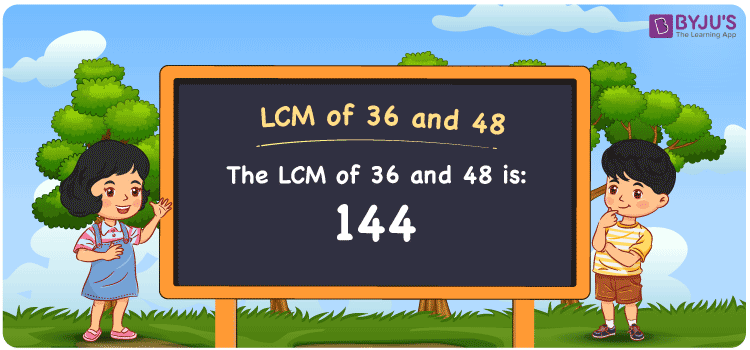Checkout JEE MAINS 2022 Question Paper Analysis : Checkout JEE MAINS 2022 Question Paper Analysis :

# LCM of 36 and 48

LCM of 36 and 48 is 144. The common multiples of the numbers 36 and 48 which are divisible evenly by the given numbers is the LCM. Least common multiples of 36 and 48 can be determined from the common multiples of the numbers given. (36, 72, 108, 144, 180, 216, ….) and (48, 96, 144, 192, 240, 288,….) are the multiples of 36 and 48. You can make use of methods such as division, prime factorisation and listing of multiples to obtain the LCM.

## What is LCM of 36 and 48?

The answer to this question is 144. The LCM of 36 and 48 using various methods is shown in this article for your reference. The LCM of two non-zero integers, 36 and 48, is the smallest positive integer 144 which is divisible by both 36 and 48 with no remainder.## How to Find LCM of 36 and 48?

LCM of 36 and 48 can be found using three methods:

• Prime Factorisation
• Division method
• Listing the multiples

### LCM of 36 and 48 Using Prime Factorisation Method

The prime factorisation of 36 and 48, respectively, is given by:

36 = 2 x 2 x 3 x 3 = 2² x 3²

48 = 2 x 2 x 2 x 2 x 3 = 2 x 3¹

LCM (36, 48) = 144

### LCM of 36 and 48 Using Division Method

We’ll divide the numbers (36, 48) by their prime factors to get the LCM of 36 and 48 using the division method (preferably common). The LCM of 36 and 48 is calculated by multiplying these divisors.

 2 36 48 2 18 24 2 9 12 2 9 6 3 9 3 3 3 1 x 1 1

No further division can be done.

Hence, LCM (36, 48) = 144

### LCM of 36 and 48 Using Listing the Multiples

To calculate the LCM of 36 and 48 by listing out the common multiples, list the multiples as shown below

 Multiples of 36 Multiples of 48 36 48 72 96 108 144 144 192

LCM (36, 48) = 144

## Video Lesson on Applications of LCM## LCM of 36 and 48 Solved Examples

Find the LCM if the product of two numbers is 1728 and the GCD is 12.

Solution:

It is given that

Product of two numbers = 1728

GCD = 12

We know that;

LCM x GCD = Product of two numbers

LCM = Product/GCD

LCM = 1728/12

LCM = 144

Hence, the LCM is 144.

## Frequently Asked Questions on LCM of 36 and 48

### Determine the LCM of 36 and 48.

The LCM of 36 and 48 is 144. The multiples of 36 and 48 and the common multiple exactly divisible by the two given numbers should be known to find the LCM.

### List the methods used to determine the LCM of 36 and 48.

The methods that can be used to determine the LCM of 36 and 48 are Prime Factorization Method, Division Method and Listing multiples.

### What is the GCF if the LCM of 36 and 48 is 144?

LCM x GCF = 36 x 48

Given

LCM of 36 and 48 = 144

144 x GCF = 1728

GCF = 1728/144 = 12